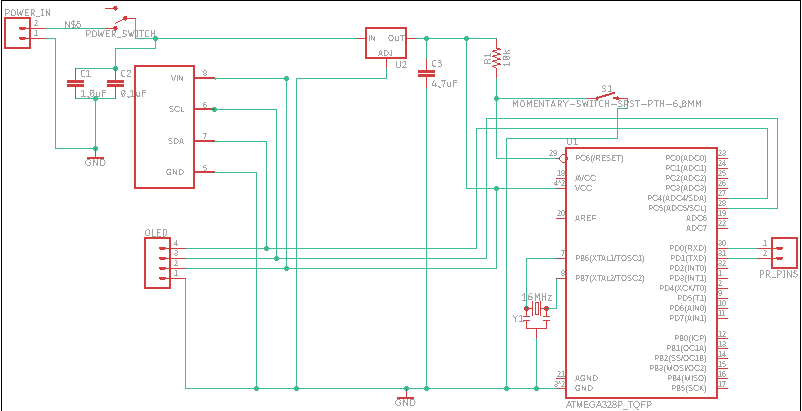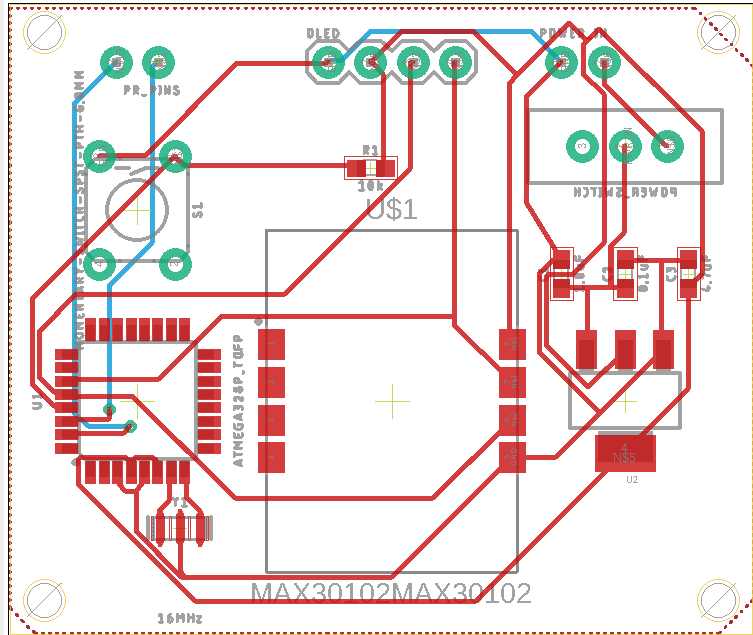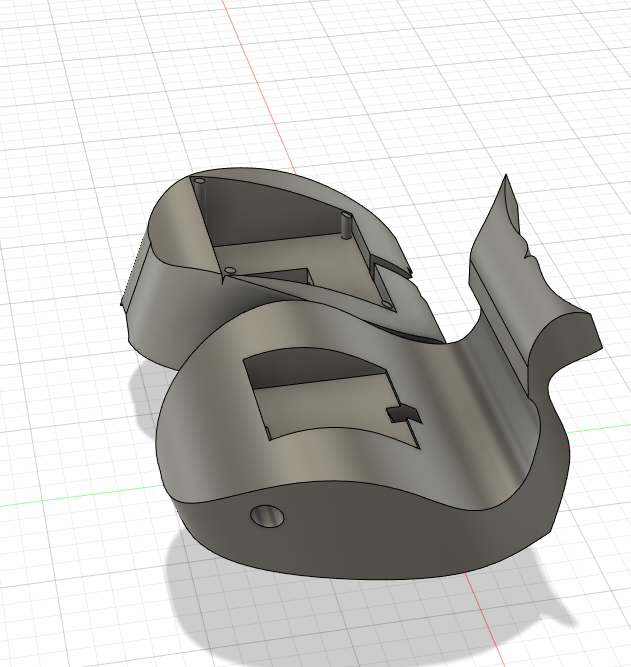CE 351 Spring 2023
Lab 2 3010 Heart Rate Monitor
Name:
3010 Heart Rate Monitor

Introduction: This lab uses a Max3010 and arduino to record heart rate and other information to an arduino and display it on an external display.

Materials: Arduino, Max3010, LCD< OLED< 10k ohm resistor, 10k ohm potentiometer, Eagle

Methods: First the 3010 was wired and the example programs were run to make sure everything worked as seen below in Figure 1.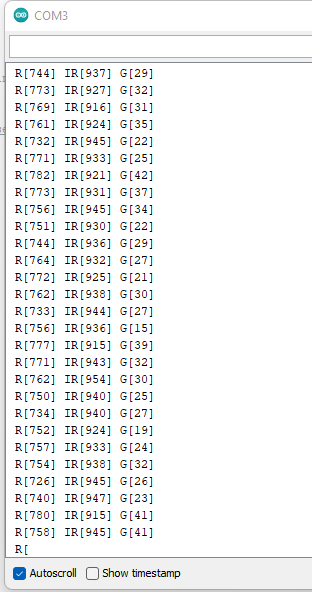Figure 1. SRed Lights, IR, and G readings from Max 3010

The readings were then plotted in the serial plotter to test the modules ability to detect when something was in front of it as seen below in Figure 2.Figure 2. Serial Plotter of IR and Red values.

BPM was then calculated using the change in IR values and SPO2 was calculated using the ratio of red to IR light as seen below in Figure 3.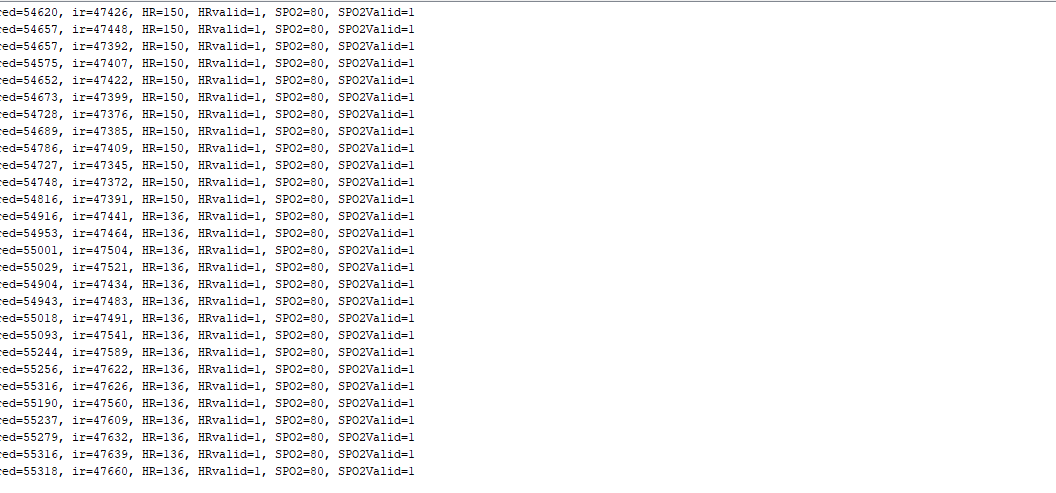Figure 3. SPo2 and Heart Rate readings

These values were then displayed on an LCD display as seen below in Figure 4.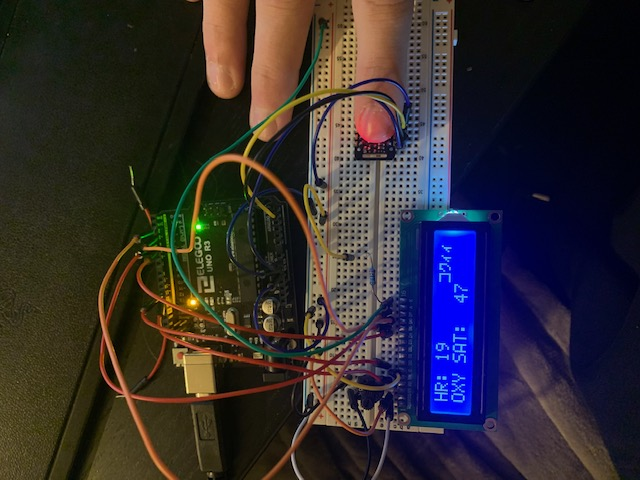Figure 4. LCD HR and OXY SAT display

An OLED display was then wired into the sytem and a program was written to display a real time pulse graph along with the HR and OXY SAT numbers as seen below in Figure 5.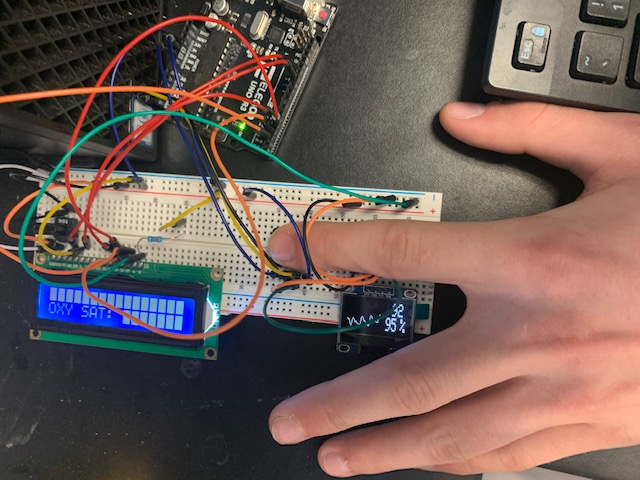An Arduino Nano was used to replace the Arduino Uno to make the system cheaper and more compact.